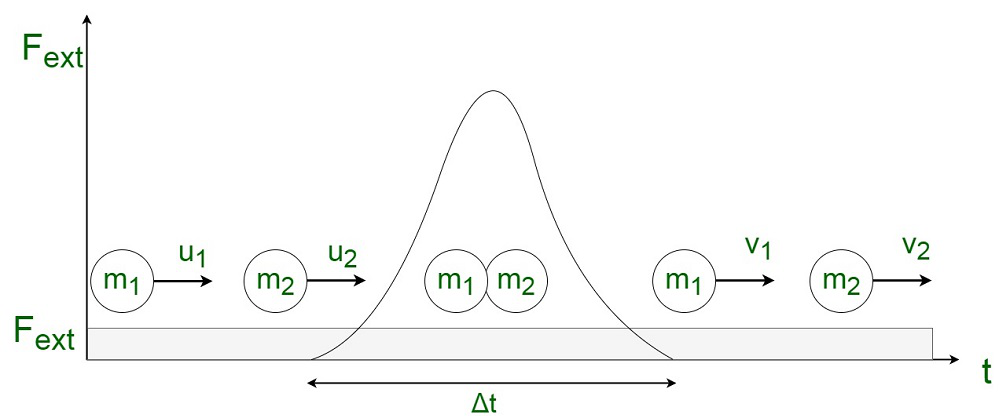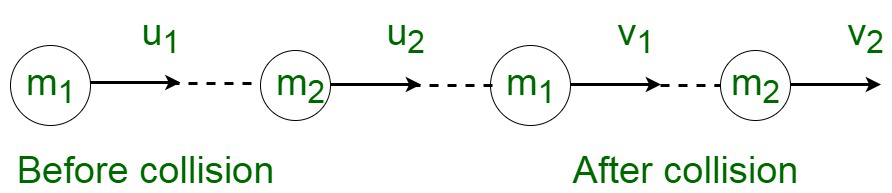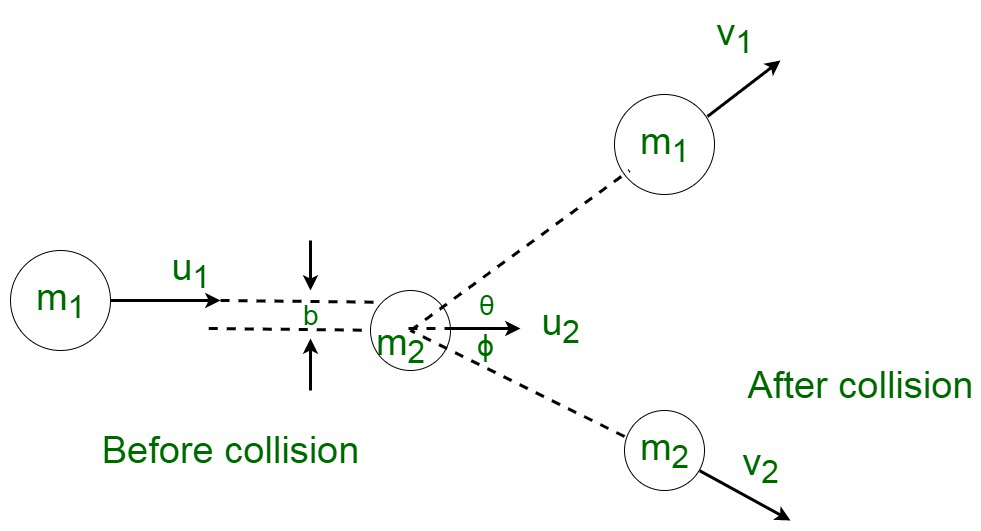# Types of Collisions

• Last Updated : 29 Jul, 2021

A body at rest continues to be at rest without any application of external force. However, when a force is applied to an object, it tends to move in the direction of the applied force, and work is then said to be done by the force. Work done by the force on any object is mathematically equivalent to the product of the force and the displacement of the object which occurs in the direction of the force.

Work done by the force is dependent only on the initial and final positions of the body, that is the displacement, and not on the actual path followed between initial and final positions. Therefore, when an object gets displaced through a small distance, say s, under the influence of a constant force F, the work done by the force is equivalent to,

W = F × s = F s cos θ

where θ is the smaller angle between F and s.

The S.I unit of work done is Joule (J). It is a scalar quantity, which has magnitude only and no direction. Its dimensional formula is [ML2T-2]

Work done by a force on any object is equivalent to zero, if

1. The body is not displaced actually, i.e., s = 0.
2. The body is displaced perpendicular to the direction of force, i.e. the angle between the force and displacement is, θ = 90°

Energy is the fundamental form of living for all living beings. The Sun in this universe is considered as the elemental form of energy on the planet of Earth. Energy refers to the ability of any object to do work. Energy is required to perform work. It is a qualitative property of a body. The law of conservation of energy states that energy can’t be created nor destroyed. It can just be transferred from one medium to another.

The S.I unit of energy is Joule (J). It is a scalar quantity, which has magnitude only and no direction. Its dimensional formula is [ML2T-2]

Power is referred to the time rate of work done by an object. Mathematically,

Power = Rate of doing work = Work Done / Time Taken

Therefore, when an object gets displaced through a small distance, say s, under the influence of a constant force F, the work done by the power is equivalent to,

P = W / T = F × s / T

But, s / t = v, velocity of the body.

Therefore,

P = F × v = F v cos θ

where θ is the smaller angle between F and v.

The S.I unit of energy is Watt (W). It is a scalar quantity, which has magnitude only and no direction since it is only a ratio of two scalar quantities. Its dimensional formula is [ML2T-3].

### What is Collision?

A collision is an event where a strong force acts for a very short span of time between two or more bodies. A collision is an isolated event. The energy and momentum of the interacting particles undergo a change as a result of the collision. The collision may occur by actual physical contact of the involved bodies, for instance, the collision between two billiard balls or a ball and bat. There may be collisions where there is no actual physical contact, for instance, the collision of the alpha particles by a nucleus.

Any collision is guided by three distinct identifiable stages, that is, before, during, and after. Before the collision, the interacting forces between the particles are zero, since the particles are independent. Also, after the collision, the force again becomes zero. During the collision, the particles come in contact with each other, therefore, the force of interaction becomes very large. The motion of the bodies is guided by the dominating forces. Since, in most of the practical cases, the magnitude of the interacting force is unknown, therefore, Newton’s second law of motion can’t be used in such cases. The initial and final velocities can be computed using the law of conservation of momentum.

For instance, consider two bodies with masses m1 and m2, moving with velocities u1 and u2. They undergo a collision due to the application of an external force Fext for a small interval of time, and then the final velocities become v1 and v2Conservation of Momentum and Energy conservation during a Collision

According to the fundamental laws of physics, certain attributes are applicable for any types of collision :

• Momentum conservation: The collision takes place for a very small interval of time, and during this period the average impulsive force causing the collision is significantly larger than the external force acting on the system. Therefore, during a collision, the application of external forces, such as frictional or gravitational forces are not considered into account. This impulsive force is internal in nature, therefore, the total momentum of the system remains constant for all practical purposes. Therefore, it remains conserved throughout the system.
• Energy conservation: According to the law of conservation of energy, energy can neither be destroyed nor created. It can only be transferred from one medium to another. Therefore, the total energy during a collision always remains conserved. Total energy includes all the plausible forms of energy created and destroyed during a collision, such as mechanical energy, internal energy, excitation energy as well as mass-energy.

### Types of collision

During the collision, the interacting bodies come in immediate contact with each other due to which they exert forces. This activity takes place for a very short period of time. There are two types of collisions, namely :

• On the basis of conservation of kinetic energy
1. Perfectly elastic collision
2. Inelastic collision
3. Perfectly inelastic collision
• On the basis of the direction of colliding bodies
2. Oblique collision

Let us assume two bodies of masses m1 and m2 moving with initial velocities u1 and u2 in the same direction and they collide such that after collision their final velocities are v1 and v2 respectively.According to law of conservation of momentum

m1u1 + m2u2 = m1v1 + m2v2               …………(1)

⇒ m1(u1 – v1) = m2(v2 – u2)                  ………..(2)

According to law of conservation of kinetic energy, we have,……..(3)

⇒………(4)

Dividing equation (4) by equation (2)

v1 + u1 = v2+ u2    ……….(5)

⇒ u1 – u2 = v2 – v1          ………..(6)

We know,

Relative velocity of approach = Relative velocity of separation

Deriving from equation (5) we get

v2 = v1 + u1– u2

Substituting this value of  v2 in equation (i) and rearranging we get…….(7)

Similarly,……….(8)

### Perfectly Elastic Oblique CollisionLet us assume two bodies to be in motion,

By the law of conservation of momentum,

We have,

Along x-axis,

m1u2 + m2u2 = m1v1 cosθ + m2v2 cosϕ                   ……..(1)

Along y-axis,

0 = m1v1 sinθ – m2v2 sinϕ                                  ………(2)

We know,

By the law of conservation of kinetic energy  :……..(3)

### Solved Problems

Problem 1: Let us assume that, s small balls, each of mass m hit a surface elastically each second with a velocity u m/s. Calculate the force witnessed by the surface.

Solution:

Since, there is a rebound of the ball with unmodified velocity, therefore,

The change in velocity = 2u

Mass colliding with the surface =  sm

Force experienced by the surface is given by,Problem 2: Initially, a car of mass 400 kg traveling with a speed of 72 kmph accidentally crashes into a car of mass 4000 kg. Before the collision, the truck was traveling at a speed of 9 kmph, in the same direction as that of the car. As a result of the collision, the car retorts back with a speed of 18 kmph. Calculate the velocity with which the truck is moving after the collision.

Solution:

By the law of conservation of linear momentum, we have,

The summation of initial momentum is equivalent to the summation of final momentum.

m1u1 + m1u2 = m1v1 + m2v2

⇒ 400 × 72 + 4000 × 9 = 400 × (-18) + 4000 × v2

⇒ v2 = 18 km/h

Therefore, the final velocity of the car is 18 km/h.

Problem 3: A crate is kept at the origin of mass equivalent to 5 kg. A force of 10 N is applied to it to simulate its movement at an angle of 60° with the x-axis. As a result of this displacement, the crate moves by 4 m. What is the work done by the application of the force?

Solution:

Work done is given by the dot product of force and displacement, that is,

F.s = F s cos θ

=10 × 4 × cos 60°

= 20 J

Problem 4: A crate is kept on a table of mass equivalent to 1 kg. It is dragged along the horizontal table through the length of 1 m by an external force of 8 N. It is then subjected to a vertical height of 2 m. Compute the net work done.

Solution:

Work done to displace the crate horizontally , W

= F × s

= 8 × 1 = 8 J

Work done to raise the crate vertically, Wv =  F × s

= m x g x h

= 1 × 10 × 2 = 20 J

Therefore,

Net work done on the crate is WH + W

= 8 +20 = 28 J

Problem 5: A horizontal force of 5 N is required to maintain a velocity of 2 m/s for a block of 10 kg mass sliding over a rough surface. The work done by this force in one minute is?

Solution:

Mathematically,

Work done by an object = Force × displacement

⇒ W = F × s

Since, we know,

s = v x t

= F × v × t

= 5 × 2 × 60

= 600 J

My Personal Notes arrow_drop_up
Recommended Articles
Page :